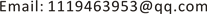1. 引言

2. 理论分析

y i t = α + x i t β + μ i t ,     i = 1 , 2 , ⋯ , N ;   t = 1 , 2 , ⋯ , T

y i t = α i + x i t β + μ i t ,     i = 1 , 2 , ⋯ , N ;   t = 1 , 2 , ⋯ , T

y i t = α i + x i t β i + μ i t ,     i = 1 , 2 , ⋯ , N ;   t = 1 , 2 , ⋯ , T

3. 实证研究3.1. 数据来源与处理

Container throughput and GDP related analysis result
GDP吞吐量
GDP1.0000000.896193

White test result
F统计量16.17248Prob. F(2,118)0.0000
Obs*R-squared26.03174Prob. Chi-Square(2)0.0000
Scaled explained SS25.64143Prob. Chi-Square(2)0.0000

3.2. 面板数据单位根检验

3.3. 面板数据协整分析

3.4. 面板数据Granger因果检验

Panel data unit root test result

LLC检验−9.17565 (0.0000)−10.434 (0.0000)

Johansen panel cointegration test result

0个协整向量124.0 (0.0000)82.72 (0.0000)

Kao test and Pedroni test result

3.5. 面板数据估计模型选择

H 1 : β 1 = β 2 = ⋯ = β N

H 2 : α 1 = α 2 = ⋯ = α N ;   β 1 = β 2 = ⋯ = β N

F检验，运用R语言计算F值，F = 33.427，p-value < 2.2e−16，拒绝H2，即拒绝混合回归模型。下面检验是否接受H1，F = −426,780，拒绝H1，故选择随机效应模型。

3.6. 面板数据模型估计结果

4. 结论

Granger causality test result

LnC不是LnG Granger原因2.970230.0561拒绝
LnG不是LC Granger原因3.471500.0351拒绝

Relationship between container throughput and economic growt

0.986710.920471.105131.027360.768390.74916

0.638950.272480.69743−0.341991.42437